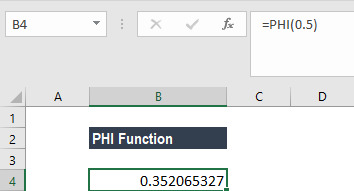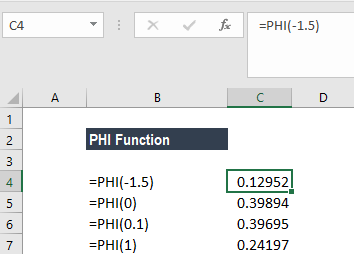# PHI Function

Returns the value of the density function for a standard normal distribution for a supplied number

## What is the PHI Function?

The PHI function is an Excel Statistical function. It will return the value of the density function for a standard normal distribution for a supplied number. The function was introduced in MS Excel 2013 and hence unavailable in earlier versions.

### Formula

=PHI(x)

The PHI function uses the following argument:

1. X (required argument) – This is the number for which we want the density of the standard normal distribution.

### How to use PHI Function in Excel?

To understand the uses of the function, let’s consider a few examples:

#### Example 1

Suppose we want to find the value of the density function for a standard normal distribution. The formula to use is =PHI(0.5).

We get the result below:#### Example 2

The worksheet below shows the Excel PHI function, which is used to evaluate the function for four different values of x.We can prepare a normal distribution chart based on the results obtained from the function.

### Things to remember about the PHI Function

1. #VALUE! error – Occurs when x uses a data type that is not valid, such as a non-numeric value.
2. #NUM! error – Occurs when x is a numeric value that is not valid.

Thanks for reading CFI’s guide to important Excel functions! By taking the time to learn and master these functions, you’ll significantly speed up your financial analysis. To learn more, check out these additional CFI resources:

• Excel Functions for Finance